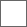• 14回复贴，共1

### C#基础复习

C# 定义常量和声明变量的区分

C# 数据类型
char 字符 引号 例子：char sex='男‘
string 字符串 双引号 例子： string sex=“男”
int 整数 例子：int age=21 ]
double 小数 例子：double salary=21.1
C#类型转化：

C#的运算符：

①交换两个变量：例子21→12

a=21 b=12
int temp
temb=a
a=b
b=temp

namespace Test

{
class Program
{
static void Main(string[] args)
{
int[] age = new int[] ;//声明并初始化长度为4的整形数组
//为数组元素赋值
age[ ] = 18;
age[ ] = 20;
age[ ] = 23;
age[ ] = 17;
Console.WriteLine("四名同学的年龄是：{0}、{1}、{2}、{3}",
age[ ],age[ ],age[ ],age[ ]);
}
}
}

string text; if (year % 4 == 0) { text = "闰年"; } else { text = "平年";}

string text = year % 4 == 0 ? "闰年" : "平年";

switch 条件结构，主要用来对变量进行多个分支的等值判断。
switch (变量){case1：分支1; break;
case2：分支2; break;
case3：分支1; break;
default:分支1; break; }
（变量）与每一个 case 后面的常量进行等值比较，如果相等，就执行对应的分支。执行分支以后， break 关键字会使 switch 结构中止，不会再判断后面的常量。如果变量与所有的常量都不相同，则执行 default 后面的分支。

{
int x = 1;
int sum = 0;//和，初始化为0
while (x <= 30)//循环条件
{
if (x%2==1)//筛选条件
sum += x;
x++;
}
Console.Write("1-30奇数的和："+sum);
}for (int x = 1; x < 10; x++)

{
if(x==3||x==8)continue;
Console.Write(x);
}{
int[] age = new int  ;//声明并初始化长度为4的整形数组
//为数组元素赋值
age[ 0] = 18;
age[ 1] = 20;
age[ 2] = 23;
age[ 3] = 17;
Console.WriteLine("四名同学的年龄是：{0}、{1}、{2}、{3}",
age[0 ],age[1 ],age[ 2],age[ 3]);
}
}
}

static void Main(string{}args)
string [ ]names=new string[ 3];
names="段菲";
names="段乔";
names="段钰";
for(i=0,i<names.lenth,i++,){
Console.Write=9(names.lenth)
}

static void Main(string{}args)
string [ ]names=new string[ 3];
names="段菲";
names="段乔";
names="段钰";
for(int i=0,i<names.lenth,i++,)
{
Console.Write=9(names[i]+''')
}

int[] score = new int[] {89,39,100,51,94,65,70 };//分数
Console.Write("不及格的有：");
for (int i = 0; i < score.Length ; i++)
{
if( score[i]<60)//筛选条件
Console.Write(score[i]+",");
}

int[] score = { 85,76,98,100,62,60};//分数
bool hasNopass = false;//记录是否有不及格的，默认没有
for (int i = 0; i < score.Length; i++)
{
if (score[i] < 60)//如果有不及格的
{
hasNopass = true;
break;//记录有不及格的

}
}
if (hasNopass)
Console.WriteLine("有人不及格");
else
Console.WriteLine("都及格啦！");
}

int[,] arr = new int[2,3]; //包含2个一维数组，每个一维数组包含3个变量，总共2*3=6个数组元素
arr[1,0] = 28; //二维数组元素有2个索引，都是从0开始，以arr数组为例，元素索引从[0,0]到[1,2]
Console.Write( arr[1,0] );using System;
using System.Collections.Generic;
using System.Linq;
using System.Text;
namespace 验证用户登录信息

{
class Program
{
static void Main(string[] args)
{
string names = " ";
int cishu = 3;
Random a = new Random();
int b = a.Next(1000, 10000);
int c = a.Next(1000, 10000);
int d = a.Next(1000, 10000);
int e = a.Next(1000, 10000);
int f = a.Next(1000, 10000);
int g = a.Next(1000, 10000);
int h = a.Next(1000, 10000);
int l = a.Next(1000, 10000);
bool q = true;

while (q)
{
bb: Console.WriteLine("\n------------------------------主菜单-------------------------------");
Console.WriteLine("\n\t\t\t欢迎使用丿东灬东客户端");
Console.WriteLine("\n\t\t\t\t1、注册\n\n\t\t\t\t2、登陆\n\n\t\t\t\t3、退出");
Console.Write("\n请选择:");
Console.Clear();
switch (xuan)
{
case 1:
Console.WriteLine("\n---------------------------------注册--------------------------------");
Boolean i = true;
while (i)
{
Console.Write("\n请输入用户名：");

names = name1;
Console.Write("\n请输入密码:");
Console.Write("\n确认密码:");
{
Console.Write("\n密码不一致，请从新输入。");
i = true;
}
else
{
Console.WriteLine("\n注册成功！！即将返回主菜单.....");
i = false;
Console.Clear();
}
};
break;
case 2:
Console.WriteLine("\n---------------------------------登录--------------------------------");
q = false;
Boolean n = true;
while (n)
{
Console.Write("\n用户名：");
Console.Write("\n密码：");
{
Console.WriteLine("\n登陆成功！正在加载中......");
Console.Clear();
n = false;
}
else
{
cishu--;
if (cishu != 0)
{
Console.WriteLine("\n密码或用户名,您还有{0}次输入机会！继续登陆请输入（y）,否则返回", cishu);
}
if (cishu == 0)
{
Console.WriteLine("您的次数已经用尽！请休息一下！");
goto aa;
break;
}
Console.Clear();
if (jd == "y")
{

}
else
{
Console.Clear();
goto bb;
}
n = true;
}
}
break;
case 3:
q = false;
goto aa;
break;
default:
Console.WriteLine("\n您输入的编号有误，请检查!");

break;

}

}
for (int i = 0; i < 2; )
{

Console.WriteLine("\t\t\t欢迎使用丿东灬东网络,祝您使用愉快！");
Console.WriteLine("----------------------------------------------------------------------");
Console.WriteLine("\t\t\t1、信息编辑\n\n\t\t\t2、管理账号\n\n\t\t\t3、注销\n\n\t\t\t4、抽奖\n\n\t\t\t5、退出\n");
Console.Write("请选择：");
switch (xz)
{
case 1:
Console.WriteLine("\n---------------------------------信息管理--------------------------------");
Console.Write("\n您的姓名：");
Console.Write("\n您的性别：");
Console.Write("\n您的生日");
Console.WriteLine("----------------------------------------------------------------------");
Console.WriteLine("您的信息已经修改成以下内容：\n姓名:{0}\n性别：{1}\n生日：{2}", ming, sex, age);
Console.WriteLine("----------------------------------------------------------------------");
break;
case 2:
Console.WriteLine("\n---------------------------------账号管理--------------------------------");
for (int j = 0; j < 2; )
{
Console.WriteLine("\n您的用户名：" + names);
Console.WriteLine("如果修改请输入y，否则请输入n");
if (yn == "y")
{
Console.Write("\n您的新用户名：");
Console.Write("\n您的新密码：");
Console.WriteLine("修改成功！！！");
}
else
{
j = 2;
}
}
break;
case 3:
Console.WriteLine("\n感谢使用;");
i = 2;
break;
case 5:
Console.WriteLine("\n期待您的下次使用；");
i = 2;
break;
case 4:
Console.Write("\n请输入您的会员账号(四位数字):");

Console.Write("\n\n本期的幸运数字为：{0} {1} {2} {3}", b, c, d, e,f,g,h,l);
if (id == b || id == c || id == d || id == e||id==f||id==g||id==h||id==l)
{
Console.WriteLine("\n\n恭喜您中奖了；中奖码为：(8523698) :请牢记！");
}
else
{
Console.WriteLine("\n\n对不起您没有中奖!!!\n");
}
break;
default:
Console.WriteLine("\n您的输入有误，请检查");
break;
};

}
aa: Console.WriteLine("\n按回车关闭系统！！！");
}
}
}

namespace
{
class Program
{
static void Main(string[] args)
{
Console.Write("请输入直角三角形的行数：");
string xing = "*";
string kong = " ";
int m = 1;
int q =0;
for (int i = 0; i <h ; i++)
{
for (int j = 0; j < m; j++)
{
Console.Write(xing);
}
Console.WriteLine();
m++;
}
Console.Write("请输入等腰三角形的行数：");
m = 1;
for (int i = 0; i < dy; i++)
{
for (int j =0; j < dy-1-q ; j++)
{
Console.Write(kong);
}
for (int n= 0; n <m; n++)
{
Console.Write(xing);
};
m+=2;
q++;
Console.WriteLine();
}
int ji=0;
q = 0;
int w=1;
Console.Write("请输入菱形行数：");
for (int i = 0; i < 1; ) {
Console.Write("请输入奇数：");
if(ji%2==0){

}else{
i=1;
}
}
for (int i = 0; i <hang*2-1; i++)
{
for (int j = 0; j < ji-1-q; j++)
{
Console.Write(kong);
}
for (int n = 0; n <w ; n++)
{
Console.Write(xing);
}
if (i >= hang-1) {
w -= 2;
q--;
}
else
{
w += 2;
q++;
};
Console.WriteLine();
}

}
}
}

static void Main(string[] args)
{
int a;
int b;
int c;
Console.WriteLine("请输入a值：");
Console.WriteLine("请输入b值：");
Console.WriteLine("请输入c值：");
if (a < b && b < c)
{
Console.WriteLine("a<b<c");

}
if (a < c && c < b)
{
Console.WriteLine("a<c<b");

}
if (b < a && a < c)
{
Console.WriteLine("b<a<c");

}
if (b < c && c < a)
{
Console.WriteLine("b<c<a");

}
if (c < b&& b < a)
{
Console.WriteLine("c<b<a");

}
if (c < a && a < b)
{
Console.WriteLine("c<a<b");

}